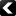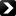Under the auspices of the Computational Complexity Foundation (CCF)LATEST > REPORTS:PreviousNextTR23-142 | 21st September 2023
Tom Gur, Wilfred Salmon, Sergii Strelchuk

#### Provable Advantage in Quantum PAC Learning

We revisit the problem of characterising the complexity of Quantum PAC learning, as introduced by Bshouty and Jackson [SIAM J. Comput.
1998, 28, 1136–1153]. Several quantum advantages have been demonstrated in this setting, however, none are generic: they apply to particular concept classes and typically only work when the distribution ... more >>>

TR23-141 | 19th September 2023

#### A Tight Lower Bound of $\Omega(\log n)$ for the Estimation of the Number of Defective Items

Let $X$ be a set of items of size $n$ , which may contain some defective items denoted by $I$, where $I \subseteq X$. In group testing, a {\it test} refers to a subset of items $Q \subset X$. The test outcome is $1$ (positive) if $Q$ contains at least ... more >>>

TR23-140 | 20th September 2023
Eshan Chattopadhyay, Jesse Goodman, Mohit Gurumukhani

#### Extractors for Polynomial Sources over $\mathbb{F}_2$

We explicitly construct the first nontrivial extractors for degree $d \ge 2$ polynomial sources over $\mathbb{F}_2^n$. Our extractor requires min-entropy $k\geq n - \frac{\sqrt{\log n}}{(d\log \log n)^{d/2}}$. Previously, no constructions were known, even for min-entropy $k\geq n-1$. A key ingredient in our construction is an input reduction lemma, which allows ... more >>>PreviousNextISSN 1433-8092 | Imprint